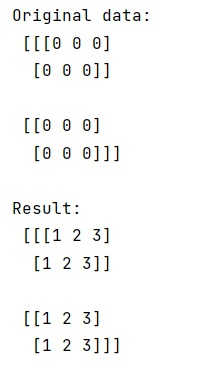# numpy.full_like() Method with Example

Learn about the numpy.full_like() method with its usage, syntax, and example.
Submitted by Pranit Sharma, on March 27, 2023

## Description and Usage

The numpy.full_like() method returns a new array with the same shape and type as the given array and fills the array with the "fill_value" value. It is like numpy.full() which returns a new array of a given shape and type and it fills the array with the "fill_value" value.

## Syntax

```numpy.full_like(a, fill_value, dtype=None, order='K', subok=True, shape=None)
```

## Parameter(s)

• a: array-like value, the shape and type of a will be the shape of the type of the output.
• fill_value: The values which need to be filled in the array
• dtype: It is optional, if given, it overrides the default data type
• order: It is an optional parameter and used to override the memory layout of the result.
• subok: An optional and Bool type parameter. If we set it to True, then newly created array will use the sub-class type of a, otherwise it will be a base-class array.
• shape: An optional and int or sequence of ints type. It is used to override the shape of the result.

## Return Value

This method returns an Array of fill_value with the same shape and type as a.

## Example: Python code to demonstrate the example of numpy.full_like()

```# Import numpy
import numpy as np

# Creating a numpy array
arr = np.zeros([2, 2, 3], dtype=int)

# Display original data
print("Original data:\n",arr,"\n")

# Creating a full array like arr
res = np.full_like(arr, [1, 2, 3])

# Display result
print("Result:\n",res)
```

### Outputnumpy.full_like